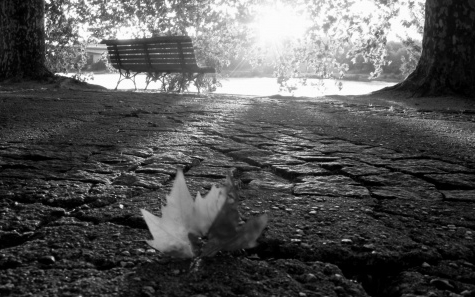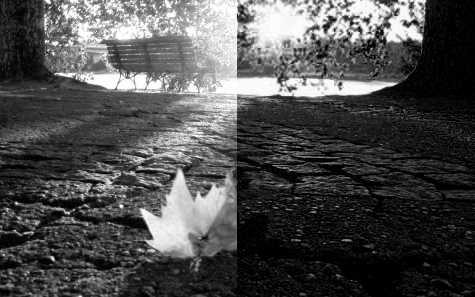### Image Grayscaling Algorithms (with Java Code)

You may definitely have used tools like Photoshop and GIMP to convert images into gray-scale in a single click. Even most programming languages have built-in functions for the task but how does it happen on the pixel level?

Here are a few gray-scaling algorithms:The Original Image

1) Averaging

Averaging is a quick gray-scaling method which returns a not-so-good but a usable image as its output. It takes the average of the Red, Green and Blue values of the pixels and sets the R,G,B to the averaged value.

Gray = (Red + Blue + Green)/3
Red = Gray
Green = Gray
Blue = GrayAveraged

2) Luma (Correction for human eye)

The human eye perceives different colors differently. It sees Green more than Red and Red more than Blue. Hence to get a image corrected to how we view us we should take a weighted averaged of the RGB values with Green > Red > Blue. There's another debate about the best weights for each color in the average. I enlist a few here.

Gray = (Red*0.3 + Green*0.59 + Blue*0.11)
Gray = (Red*0.2126 + Green*0.7152 + Blue*0.722)
Gray = (Red*0.299 + Green*0.587 + Blue*0.114)(Red*0.3 + Green*0.59 + Blue*0.11)

3) Desaturation

Other than RGB one representation of image mode is HSL (Hue, Saturation, Lightness) where hue means the color (i.e. Red, Green, Yellow, Orange etc.), Saturation is how vivid the color is (gray has 0 saturation) and lightness is the brightness of the color (white has full lightness). In desaturation we bring down the S (saturation) value of each pixel to zero. The simplest way to achieve this in the RGB model is,

Gray = (maximum(R,G,B) + minimum(R,G,B))/2Desaturated

4) Decomposition

In desaturation either the maximum or the minimum of R,G,B value is used as the Gray value for each pixel of image.

Gray = maximum(R,G,B)
or
Gray = minimum(R,G,B)Left: Max, Right: Min

5) Single Channel Gray-scaling

In this method we use the value of either one of the R/G/B value as the gray value for every pixel. Though this method may sound ridiculous it is actually used in many cameras to take gray-scale shots. Generally Green value is used for obvious reasons.

Gray = Red
or
Gray = Green
or
Gray = BlueSingle Channel: R|G|B
Here is the Java code for performing these operations:

```import java.io.File;
import javax.imageio.ImageIO;
import java.awt.image.BufferedImage;
import java.io.IOException;
import java.awt.Color;
import static java.lang.System.out;
public class Grayscaler
{
public static String ImageName="raw.png";

public static void main(String args[])throws IOException
{
// Image objects to store all the types of gray-scaled images
BufferedImage image,averaged,luma,desaturated,decoMax,decoMin,channelR,channelG,channelB;

int WIDTH = image.getWidth();
int HEIGHT = image.getHeight();

averaged = new BufferedImage(WIDTH,HEIGHT,image.getType());
luma = new BufferedImage(WIDTH,HEIGHT,image.getType());
decoMax = new BufferedImage(WIDTH,HEIGHT,image.getType());
decoMin = new BufferedImage(WIDTH,HEIGHT,image.getType());
desaturated = new BufferedImage(WIDTH,HEIGHT,image.getType());
channelR = new BufferedImage(WIDTH,HEIGHT,image.getType());
channelG = new BufferedImage(WIDTH,HEIGHT,image.getType());
channelB = new BufferedImage(WIDTH,HEIGHT,image.getType());

// Looping through each pixel
for(int y=0;y<HEIGHT;y++)
{
for(int x=0;x<WIDTH;x++)
{
int RGB = image.getRGB(x,y);
int R = (RGB >> 16) & 0xff; // Red Value
int G = (RGB >> 8) & 0xff;	// Green Value
int B = (RGB) & 0xff;		// Blue Value

// Getting the max of RGB
int MAX = Math.max(R,G);
MAX = Math.max(MAX,B);

// Getting the min of RGB
int MIN = Math.min(R,G);
MIN = Math.min(MIN,B);

// Averaging
int GRAY = (R+G+B)/3;
Color color = new Color(GRAY,GRAY,GRAY);
averaged.setRGB(x,y,color.getRGB());

// Human Eye Correction
GRAY = (int)(R*0.3f + G*0.59f + B*0.11f);
color = new Color(GRAY,GRAY,GRAY);
luma.setRGB(x,y,color.getRGB());

// Desaturation
GRAY = (MAX + MIN)/2;
color = new Color(GRAY,GRAY,GRAY);
desaturated.setRGB(x,y,color.getRGB());

// Decomposition - MAX
color = new Color(MAX,MAX,MAX);
decoMax.setRGB(x,y,color.getRGB());

// Decomposition - MIN
color = new Color(MIN,MIN,MIN);
decoMin.setRGB(x,y,color.getRGB());

// Single Channel Gray-Scaling - Red
color = new Color(R,R,R);
channelR.setRGB(x,y,color.getRGB());

// Single Channel Gray-Scaling - Green
color = new Color(G,G,G);
channelG.setRGB(x,y,color.getRGB());

// Single Channel Gray-Scaling - Blue
color = new Color(B,B,B);
channelB.setRGB(x,y,color.getRGB());
}
}

// Saving the Images
ImageIO.write(averaged,"PNG",new File("averaged.png"));
ImageIO.write(luma,"PNG",new File("luma.png"));
ImageIO.write(desaturated,"PNG",new File("desaturated.png"));
ImageIO.write(decoMax,"PNG",new File("decoMax.png"));
ImageIO.write(decoMin,"PNG",new File("decoMin.png"));
ImageIO.write(channelB,"PNG",new File("ChannelB.png"));
ImageIO.write(channelG,"PNG",new File("ChannelG.png"));
ImageIO.write(channelR,"PNG",new File("ChannelR.png"));
}
}
```